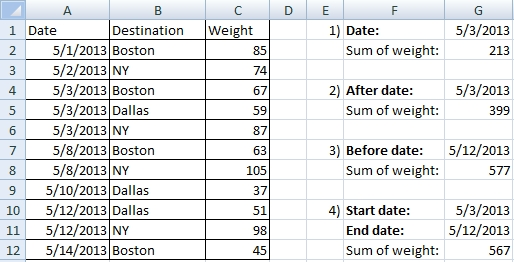# SUMIF and COUNTIF with date

Date can be used as a condition in the Excel functions SUMIF and COUNTIF. But there can be some problems. I show you examples.## SUMIF for the exact date

Example No. 1

You have two choices:

1) You can use a formula with the cell address.

=SUMIF(A2:A12,G1,C2:C12)

2) You can write the date right into the formula. The date must be in the quotation marks.

=SUMIF(A2:A12,”5/3/2013″,C2:C12)

## SUMIF for data after the exact date

Example No. 2

1) You can use a formula with the cell address. The > character must be in the quotation marks and must be joined with the cell using &.

=SUMIF(A2:A12,”>”&G4,C2:C12)

2) You can write the date right into the formula. The date and > must be in the quotation marks.

=SUMIF(A2:A12,”>5/3/2013″,C2:C12)

If you need to include the start date, change the condition to >=

## SUMIF for data before the exact date

Example No. 3

1) You can use a formula with the cell address. The < character must be in the quotation marks and must be joined with the cell using &.

=SUMIF(A2:A12,”<”&G7,C2:C12)

2) You can write the date right into the formula. The date and < must be in the quotation marks.

=SUMIF(A2:A12,”<5/12/2013″,C2:C12)

If you need to include the end date, change the condition to <=

## SUMIF for data between two dates

Example No. 4

You have also two choices:

1) You can use a formula with the cell address.

=SUMIF(A2:A12,”<=”&G11,C2:C12)-SUMIF(A2:A12, “<”&G10,C2:C12)

2) You can write the date right into the formula. The date and < must be in the quotation marks.

=SUMIF(A2:A12,”<=5/12/2013″,C2:C12)-SUMIF(A2:A12,”<5/3/2013″,C2:C12)

The result includes the start and end dates.

## SUMIFS for data between two dates

Example No. 4

If you are using Excel 2007 and higher, you can use SUMIFS function:

=SUMIFS(C2:C12,A2:A12,”>=5/3/2013″,A2:A12,”<=5/12/2013″)

For SUMIFS, COUNTIF and COUNTIFS apply exactly the same principles.

1.Hello,
I am having an issue with a formula. I have a cell that counts a total based on two criteria (a group and a status bar). It works just fine, but now I would also like this cell to filter by date submitted within a fiscal year. It looks like it should work but when I test the sheet it gives me an error. Any advice? The part that is not working is the last bit.( M4:M9,”>”&C227). C227 is the date criteria and M:4:M9 is the range of dates submitted.

=SUM(COUNTIFS(\$I\$4:\$I\$153,D171,\$C\$4:\$C\$153,{“PRO BOARD”,”NON-SELECT/DECLINED E/C”,”PRO REC Y-PENDING FINSEL”,”SELECT LTR/OATH RECEIVED”,”ENLISTED/COMMISSIONED”,”SHIPPED TO NTC/COLLEGIATE”},M4:M9,”>”&C227))

•Hi,

You can try to change data format on your criteria cell (C227). Sometimes it works different when the criteria format is Date,Text or General.

2.Thanks